# Matrices¶

## Creating matrices¶

### Basic methods¶

Matrices in mpmath are implemented using dictionaries. Only non-zero values are stored, so it is cheap to represent sparse matrices.

The most basic way to create one is to use the matrix class directly. You can create an empty matrix specifying the dimensions:

>>> from mpmath import *
>>> mp.dps = 15; mp.pretty = False
>>> matrix(2)
matrix(
[['0.0', '0.0'],
['0.0', '0.0']])
>>> matrix(2, 3)
matrix(
[['0.0', '0.0', '0.0'],
['0.0', '0.0', '0.0']])


Calling matrix with one dimension will create a square matrix.

To access the dimensions of a matrix, use the rows or cols keyword:

>>> A = matrix(3, 2)
>>> A
matrix(
[['0.0', '0.0'],
['0.0', '0.0'],
['0.0', '0.0']])
>>> A.rows
3
>>> A.cols
2


You can also change the dimension of an existing matrix. This will set the new elements to 0. If the new dimension is smaller than before, the concerning elements are discarded:

>>> A.rows = 2
>>> A
matrix(
[['0.0', '0.0'],
['0.0', '0.0']])


Internally convert is applied every time an element is set. This is done using the syntax A[row,column], counting from 0:

>>> A = matrix(2)
>>> A[1,1] = 1 + 1j
>>> print A
[0.0           0.0]
[0.0  (1.0 + 1.0j)]


A more comfortable way to create a matrix lets you use nested lists:

>>> matrix([[1, 2], [3, 4]])
matrix(
[['1.0', '2.0'],
['3.0', '4.0']])


Convenient functions are available for creating various standard matrices:

>>> zeros(2)
matrix(
[['0.0', '0.0'],
['0.0', '0.0']])
>>> ones(2)
matrix(
[['1.0', '1.0'],
['1.0', '1.0']])
>>> diag([1, 2, 3]) # diagonal matrix
matrix(
[['1.0', '0.0', '0.0'],
['0.0', '2.0', '0.0'],
['0.0', '0.0', '3.0']])
>>> eye(2) # identity matrix
matrix(
[['1.0', '0.0'],
['0.0', '1.0']])


You can even create random matrices:

>>> randmatrix(2)
matrix(
[['0.53491598236191806', '0.57195669543302752'],
['0.85589992269513615', '0.82444367501382143']])


### Vectors¶

Vectors may also be represented by the matrix class (with rows = 1 or cols = 1). For vectors there are some things which make life easier. A column vector can be created using a flat list, a row vectors using an almost flat nested list:

>>> matrix([1, 2, 3])
matrix(
[['1.0'],
['2.0'],
['3.0']])
>>> matrix([[1, 2, 3]])
matrix(
[['1.0', '2.0', '3.0']])


Optionally vectors can be accessed like lists, using only a single index:

>>> x = matrix([1, 2, 3])
>>> x
mpf('2.0')
>>> x[1,0]
mpf('2.0')


### Other¶

Like you probably expected, matrices can be printed:

>>> print randmatrix(3)
[ 0.782963853573023  0.802057689719883  0.427895717335467]
[0.0541876859348597  0.708243266653103  0.615134039977379]
[ 0.856151514955773  0.544759264818486  0.686210904770947]


Use nstr or nprint to specify the number of digits to print:

>>> nprint(randmatrix(5), 3)
[2.07e-1  1.66e-1  5.06e-1  1.89e-1  8.29e-1]
[6.62e-1  6.55e-1  4.47e-1  4.82e-1  2.06e-2]
[4.33e-1  7.75e-1  6.93e-2  2.86e-1  5.71e-1]
[1.01e-1  2.53e-1  6.13e-1  3.32e-1  2.59e-1]
[1.56e-1  7.27e-2  6.05e-1  6.67e-2  2.79e-1]


As matrices are mutable, you will need to copy them sometimes:

>>> A = matrix(2)
>>> A
matrix(
[['0.0', '0.0'],
['0.0', '0.0']])
>>> B = A.copy()
>>> B[0,0] = 1
>>> B
matrix(
[['1.0', '0.0'],
['0.0', '0.0']])
>>> A
matrix(
[['0.0', '0.0'],
['0.0', '0.0']])


Finally, it is possible to convert a matrix to a nested list. This is very useful, as most Python libraries involving matrices or arrays (namely NumPy or SymPy) support this format:

>>> B.tolist()
[[mpf('1.0'), mpf('0.0')], [mpf('0.0'), mpf('0.0')]]


## Matrix operations¶

You can add and substract matrices of compatible dimensions:

>>> A = matrix([[1, 2], [3, 4]])
>>> B = matrix([[-2, 4], [5, 9]])
>>> A + B
matrix(
[['-1.0', '6.0'],
['8.0', '13.0']])
>>> A - B
matrix(
[['3.0', '-2.0'],
['-2.0', '-5.0']])
>>> A + ones(3)
Traceback (most recent call last):
File "<stdin>", line 1, in <module>
File "...", line 238, in __add__


It is possible to multiply or add matrices and scalars. In the latter case the operation will be done element-wise:

>>> A * 2
matrix(
[['2.0', '4.0'],
['6.0', '8.0']])
>>> A / 4
matrix(
[['0.25', '0.5'],
['0.75', '1.0']])
>>> A - 1
matrix(
[['0.0', '1.0'],
['2.0', '3.0']])


Of course you can perform matrix multiplication, if the dimensions are compatible:

>>> A * B
matrix(
[['8.0', '22.0'],
['14.0', '48.0']])
>>> matrix([[1, 2, 3]]) * matrix([[-6], , [-2]])
matrix(
[['2.0']])


You can raise powers of square matrices:

>>> A**2
matrix(
[['7.0', '10.0'],
['15.0', '22.0']])


Negative powers will calculate the inverse:

>>> A**-1
matrix(
[['-2.0', '1.0'],
['1.5', '-0.5']])
>>> nprint(A * A**-1, 3)
[      1.0  1.08e-19]
[-2.17e-19       1.0]


Matrix transposition is straightforward:

>>> A = ones(2, 3)
>>> A
matrix(
[['1.0', '1.0', '1.0'],
['1.0', '1.0', '1.0']])
>>> A.T
matrix(
[['1.0', '1.0'],
['1.0', '1.0'],
['1.0', '1.0']])


### Norms¶

Sometimes you need to know how “large” a matrix or vector is. Due to their multidimensional nature it’s not possible to compare them, but there are several functions to map a matrix or a vector to a positive real number, the so called norms.

mpmath.norm(ctx, x, p=2)

Gives the entrywise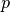-norm of an iterable x, i.e. the vector norm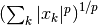, for any given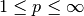.

Special cases:

If x is not iterable, this just returns absmax(x).

p=1 gives the sum of absolute values.

p=2 is the standard Euclidean vector norm.

p=inf gives the magnitude of the largest element.

For x a matrix, p=2 is the Frobenius norm. For operator matrix norms, use mnorm() instead.

You can use the string ‘inf’ as well as float(‘inf’) or mpf(‘inf’) to specify the infinity norm.

Examples

>>> from mpmath import *
>>> mp.dps = 15; mp.pretty = False
>>> x = matrix([-10, 2, 100])
>>> norm(x, 1)
mpf('112.0')
>>> norm(x, 2)
mpf('100.5186549850325')
>>> norm(x, inf)
mpf('100.0')

mpmath.mnorm(ctx, A, p=1)

Gives the matrix (operator)-norm of A. Currently p=1 and p=inf are supported:

p=1 gives the 1-norm (maximal column sum)

p=inf gives the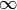-norm (maximal row sum). You can use the string ‘inf’ as well as float(‘inf’) or mpf(‘inf’)

p=2 (not implemented) for a square matrix is the usual spectral matrix norm, i.e. the largest singular value.

p='f' (or ‘F’, ‘fro’, ‘Frobenius, ‘frobenius’) gives the Frobenius norm, which is the elementwise 2-norm. The Frobenius norm is an approximation of the spectral norm and satisfies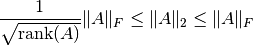The Frobenius norm lacks some mathematical properties that might be expected of a norm.

For general elementwise-norms, use norm() instead.

Examples

>>> from mpmath import *
>>> mp.dps = 15; mp.pretty = False
>>> A = matrix([[1, -1000], [100, 50]])
>>> mnorm(A, 1)
mpf('1050.0')
>>> mnorm(A, inf)
mpf('1001.0')
>>> mnorm(A, 'F')
mpf('1006.2310867787777')


## Linear algebra¶

### Decompositions¶

mpmath.cholesky(ctx, A, tol=None)

Cholesky decomposition of a symmetric positive-definite matrix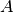. Returns a lower triangular matrix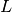such that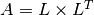. More generally, for a complex Hermitian positive-definite matrix, a Cholesky decomposition satisfying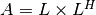is returned.

The Cholesky decomposition can be used to solve linear equation systems twice as efficiently as LU decomposition, or to test whetheris positive-definite.

The optional parameter tol determines the tolerance for verifying positive-definiteness.

Examples

Cholesky decomposition of a positive-definite symmetric matrix:

>>> from mpmath import *
>>> mp.dps = 25; mp.pretty = True
>>> A = eye(3) + hilbert(3)
>>> nprint(A)
[     2.0      0.5  0.333333]
[     0.5  1.33333      0.25]
[0.333333     0.25       1.2]
>>> L = cholesky(A)
>>> nprint(L)
[ 1.41421      0.0      0.0]
[0.353553  1.09924      0.0]
[0.235702  0.15162  1.05899]
>>> chop(A - L*L.T)
[0.0  0.0  0.0]
[0.0  0.0  0.0]
[0.0  0.0  0.0]


Cholesky decomposition of a Hermitian matrix:

>>> A = eye(3) + matrix([[0,0.25j,-0.5j],[-0.25j,0,0],[0.5j,0,0]])
>>> L = cholesky(A)
>>> nprint(L)
[          1.0                0.0                0.0]
[(0.0 - 0.25j)  (0.968246 + 0.0j)                0.0]
[ (0.0 + 0.5j)  (0.129099 + 0.0j)  (0.856349 + 0.0j)]
>>> chop(A - L*L.H)
[0.0  0.0  0.0]
[0.0  0.0  0.0]
[0.0  0.0  0.0]


Attempted Cholesky decomposition of a matrix that is not positive definite:

>>> A = -eye(3) + hilbert(3)
>>> L = cholesky(A)
Traceback (most recent call last):
...
ValueError: matrix is not positive-definite


References

### Linear equations¶

Basic linear algebra is implemented; you can for example solve the linear equation system:

  x + 2*y = -10
3*x + 4*y =  10

using lu_solve:

>>> A = matrix([[1, 2], [3, 4]])
>>> b = matrix([-10, 10])
>>> x = lu_solve(A, b)
>>> x
matrix(
[['30.0'],
['-20.0']])


If you don’t trust the result, use residual to calculate the residual ||A*x-b||:

>>> residual(A, x, b)
matrix(
[['3.46944695195361e-18'],
['3.46944695195361e-18']])
>>> str(eps)
'2.22044604925031e-16'


As you can see, the solution is quite accurate. The error is caused by the inaccuracy of the internal floating point arithmetic. Though, it’s even smaller than the current machine epsilon, which basically means you can trust the result.

If you need more speed, use NumPy, or use fp instead mp matrices and methods:

>>> A = fp.matrix([[1, 2], [3, 4]])
>>> b = fp.matrix([-10, 10])
>>> fp.lu_solve(A, b)
matrix(
[['30.0'],
['-20.0']])


lu_solve accepts overdetermined systems. It is usually not possible to solve such systems, so the residual is minimized instead. Internally this is done using Cholesky decomposition to compute a least squares approximation. This means that that lu_solve will square the errors. If you can’t afford this, use qr_solve instead. It is twice as slow but more accurate, and it calculates the residual automatically.

### Matrix factorization¶

The function lu computes an explicit LU factorization of a matrix:

>>> P, L, U = lu(matrix([[0,2,3],[4,5,6],[7,8,9]]))
>>> print P
[0.0  0.0  1.0]
[1.0  0.0  0.0]
[0.0  1.0  0.0]
>>> print L
[              1.0                0.0  0.0]
[              0.0                1.0  0.0]
[0.571428571428571  0.214285714285714  1.0]
>>> print U
[7.0  8.0                9.0]
[0.0  2.0                3.0]
[0.0  0.0  0.214285714285714]
>>> print P.T*L*U
[0.0  2.0  3.0]
[4.0  5.0  6.0]
[7.0  8.0  9.0]


The function qr computes a QR factorization of a matrix:

>>> A = matrix([[1, 2], [3, 4], [1, 1]])
>>> Q, R = qr(A)
>>> print Q
[-0.301511344577764   0.861640436855329   0.408248290463863]
[-0.904534033733291  -0.123091490979333  -0.408248290463863]
[-0.301511344577764  -0.492365963917331   0.816496580927726]
>>> print R
[-3.3166247903554  -4.52267016866645]
[             0.0  0.738548945875996]
[             0.0                0.0]
>>> print Q * R
[1.0  2.0]
[3.0  4.0]
[1.0  1.0]
>>> print chop(Q.T * Q)
[1.0  0.0  0.0]
[0.0  1.0  0.0]
[0.0  0.0  1.0]


### The singular value decomposition¶

The routines svd_r and svd_c compute the singular value decomposition of a real or complex matrix A. svd is an unified interface calling either svd_r or svd_c depending on whether A is real or complex.

Given A, two orthogonal (A real) or unitary (A complex) matrices U and V are calculated such that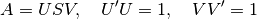where S is a suitable shaped matrix whose off-diagonal elements are zero. Here ‘ denotes the hermitian transpose (i.e. transposition and complex conjugation). The diagonal elements of S are the singular values of A, i.e. the square roots of the eigenvalues of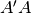or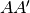.

Examples:

>>> from mpmath import mp
>>> A = mp.matrix([[2, -2, -1], [3, 4, -2], [-2, -2, 0]])
>>> S = mp.svd_r(A, compute_uv = False)
>>> print S
[6.0]
[3.0]
[1.0]
>>> U, S, V = mp.svd_r(A)
>>> print mp.chop(A - U * mp.diag(S) * V)
[0.0  0.0  0.0]
[0.0  0.0  0.0]
[0.0  0.0  0.0]


### The Schur decomposition¶

This routine computes the Schur decomposition of a square matrix A. Given A, a unitary matrix Q is determined such that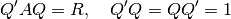where R is an upper right triangular matrix. Here ‘ denotes the hermitian transpose (i.e. transposition and conjugation).

Examples:

>>> from mpmath import mp
>>> A = mp.matrix([[3, -1, 2], [2, 5, -5], [-2, -3, 7]])
>>> Q, R = mp.schur(A)
>>> mp.nprint(R, 3)
[2.0  0.417  -2.53]
[0.0    4.0  -4.74]
[0.0    0.0    9.0]
>>> print(mp.chop(A - Q * R * Q.transpose_conj()))
[0.0  0.0  0.0]
[0.0  0.0  0.0]
[0.0  0.0  0.0]


### The eigenvalue problem¶

The routine eig solves the (ordinary) eigenvalue problem for a real or complex square matrix A. Given A, a vector E and matrices ER and EL are calculated such that

        A ER[:,i] =         E[i] ER[:,i]
EL[i,:] A         = EL[i,:] E[i]

E contains the eigenvalues of A. The columns of ER contain the right eigenvectors of A whereas the rows of EL contain the left eigenvectors.

Examples:

>>> from mpmath import mp
>>> A = mp.matrix([[3, -1, 2], [2, 5, -5], [-2, -3, 7]])
>>> E, ER = mp.eig(A)
>>> print(mp.chop(A * ER[:,0] - E * ER[:,0]))
[0.0]
[0.0]
[0.0]
>>> E, EL, ER = mp.eig(A,left = True, right = True)
>>> E, EL, ER = mp.eig_sort(E, EL, ER)
>>> mp.nprint(E)
[2.0, 4.0, 9.0]
>>> print(mp.chop(A * ER[:,0] - E * ER[:,0]))
[0.0]
[0.0]
[0.0]
>>> print(mp.chop( EL[0,:] * A - EL[0,:] * E))
[0.0  0.0  0.0]


### The symmetric eigenvalue problem¶

The routines eigsy and eighe solve the (ordinary) eigenvalue problem for a real symmetric or complex hermitian square matrix A. eigh is an unified interface for this two functions calling either eigsy or eighe depending on whether A is real or complex.

Given A, an orthogonal (A real) or unitary matrix Q (A complex) is calculated which diagonalizes A: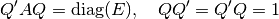Here diag(E) a is diagonal matrix whose diagonal is E. ‘ denotes the hermitian transpose (i.e. ordinary transposition and complex conjugation).

The columns of Q are the eigenvectors of A and E contains the eigenvalues:

A Q[:,i] = E[i] Q[:,i]

Examples:

>>> from mpmath import mp
>>> A = mp.matrix([[3, 2], [2, 0]])
>>> E = mp.eigsy(A, eigvals_only = True)
>>> print E
[-1.0]
[ 4.0]
>>> A = mp.matrix([[1, 2], [2, 3]])
>>> E, Q = mp.eigsy(A)                     # alternative: E, Q = mp.eigh(A)
>>> print mp.chop(A * Q[:,0] - E * Q[:,0])
[0.0]
[0.0]
>>> A = mp.matrix([[1, 2 + 5j], [2 - 5j, 3]])
>>> E, Q = mp.eighe(A)                     # alternative: E, Q = mp.eigh(A)
>>> print mp.chop(A * Q[:,0] - E * Q[:,0])
[0.0]
[0.0]


## Interval and double-precision matrices¶

The iv.matrix and fp.matrix classes convert inputs to intervals and Python floating-point numbers respectively.

Interval matrices can be used to perform linear algebra operations with rigorous error tracking:

>>> a = iv.matrix([['0.1','0.3','1.0'],
...                ['7.1','5.5','4.8'],
...                ['3.2','4.4','5.6']])
>>>
>>> b = iv.matrix(['4','0.6','0.5'])
>>> c = iv.lu_solve(a, b)
>>> print c
[  [5.2582327113062393041, 5.2582327113062749951]]
[[-13.155049396267856583, -13.155049396267821167]]
[  [7.4206915477497212555, 7.4206915477497310922]]
>>> print a*c
[  [3.9999999999999866773, 4.0000000000000133227]]
[[0.59999999999972430942, 0.60000000000027142733]]
[[0.49999999999982236432, 0.50000000000018474111]]


## Matrix functions¶

mpmath.expm(ctx, A, method='taylor')

Computes the matrix exponential of a square matrix, which is defined by the power series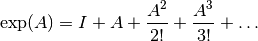With method=’taylor’, the matrix exponential is computed using the Taylor series. With method=’pade’, Pade approximants are used instead.

Examples

Basic examples:

>>> from mpmath import *
>>> mp.dps = 15; mp.pretty = True
>>> expm(zeros(3))
[1.0  0.0  0.0]
[0.0  1.0  0.0]
[0.0  0.0  1.0]
>>> expm(eye(3))
[2.71828182845905               0.0               0.0]
[             0.0  2.71828182845905               0.0]
[             0.0               0.0  2.71828182845905]
>>> expm([[1,1,0],[1,0,1],[0,1,0]])
[ 3.86814500615414  2.26812870852145  0.841130841230196]
[ 2.26812870852145  2.44114713886289   1.42699786729125]
[0.841130841230196  1.42699786729125    1.6000162976327]
[ 3.86814500615414  2.26812870852145  0.841130841230196]
[ 2.26812870852145  2.44114713886289   1.42699786729125]
[0.841130841230196  1.42699786729125    1.6000162976327]
>>> expm([[1+j, 0], [1+j,1]])
[(1.46869393991589 + 2.28735528717884j)                        0.0]
[  (1.03776739863568 + 3.536943175722j)  (2.71828182845905 + 0.0j)]


Matrices with large entries are allowed:

>>> expm(matrix([[1,2],[2,3]])**25)
[5.65024064048415e+2050488462815550  9.14228140091932e+2050488462815550]
[9.14228140091932e+2050488462815550  1.47925220414035e+2050488462815551]


The identity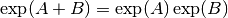does not hold for noncommuting matrices:

>>> A = hilbert(3)
>>> B = A + eye(3)
>>> chop(mnorm(A*B - B*A))
0.0
>>> chop(mnorm(expm(A+B) - expm(A)*expm(B)))
0.0
>>> B = A + ones(3)
>>> mnorm(A*B - B*A)
1.8
>>> mnorm(expm(A+B) - expm(A)*expm(B))
42.0927851137247

mpmath.cosm(ctx, A)

Gives the cosine of a square matrix, defined in analogy with the matrix exponential.

Examples:

>>> from mpmath import *
>>> mp.dps = 15; mp.pretty = True
>>> X = eye(3)
>>> cosm(X)
[0.54030230586814               0.0               0.0]
[             0.0  0.54030230586814               0.0]
[             0.0               0.0  0.54030230586814]
>>> X = hilbert(3)
>>> cosm(X)
[ 0.424403834569555  -0.316643413047167  -0.221474945949293]
[-0.316643413047167   0.820646708837824  -0.127183694770039]
[-0.221474945949293  -0.127183694770039   0.909236687217541]
>>> X = matrix([[1+j,-2],[0,-j]])
>>> cosm(X)
[(0.833730025131149 - 0.988897705762865j)  (1.07485840848393 - 0.17192140544213j)]
[                                     0.0               (1.54308063481524 + 0.0j)]

mpmath.sinm(ctx, A)

Gives the sine of a square matrix, defined in analogy with the matrix exponential.

Examples:

>>> from mpmath import *
>>> mp.dps = 15; mp.pretty = True
>>> X = eye(3)
>>> sinm(X)
[0.841470984807897                0.0                0.0]
[              0.0  0.841470984807897                0.0]
[              0.0                0.0  0.841470984807897]
>>> X = hilbert(3)
>>> sinm(X)
[0.711608512150994  0.339783913247439  0.220742837314741]
[0.339783913247439  0.244113865695532  0.187231271174372]
[0.220742837314741  0.187231271174372  0.155816730769635]
>>> X = matrix([[1+j,-2],[0,-j]])
>>> sinm(X)
[(1.29845758141598 + 0.634963914784736j)  (-1.96751511930922 + 0.314700021761367j)]
[                                    0.0                  (0.0 - 1.1752011936438j)]

mpmath.sqrtm(ctx, A, _may_rotate=2)

Computes a square root of the square matrix, i.e. returns a matrix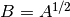such that. The square root of a matrix, if it exists, is not unique.

Examples

Square roots of some simple matrices:

>>> from mpmath import *
>>> mp.dps = 15; mp.pretty = True
>>> sqrtm([[1,0], [0,1]])
[1.0  0.0]
[0.0  1.0]
>>> sqrtm([[0,0], [0,0]])
[0.0  0.0]
[0.0  0.0]
>>> sqrtm([[2,0],[0,1]])
[1.4142135623731  0.0]
[            0.0  1.0]
>>> sqrtm([[1,1],[1,0]])
[ (0.920442065259926 - 0.21728689675164j)  (0.568864481005783 + 0.351577584254143j)]
[(0.568864481005783 + 0.351577584254143j)  (0.351577584254143 - 0.568864481005783j)]
>>> sqrtm([[1,0],[0,1]])
[1.0  0.0]
[0.0  1.0]
>>> sqrtm([[-1,0],[0,1]])
[(0.0 - 1.0j)           0.0]
[         0.0  (1.0 + 0.0j)]
>>> sqrtm([[j,0],[0,j]])
[(0.707106781186547 + 0.707106781186547j)                                       0.0]
[                                     0.0  (0.707106781186547 + 0.707106781186547j)]


A square root of a rotation matrix, giving the corresponding half-angle rotation matrix:

>>> t1 = 0.75
>>> t2 = t1 * 0.5
>>> A1 = matrix([[cos(t1), -sin(t1)], [sin(t1), cos(t1)]])
>>> A2 = matrix([[cos(t2), -sin(t2)], [sin(t2), cos(t2)]])
>>> sqrtm(A1)
[0.930507621912314  -0.366272529086048]
[0.366272529086048   0.930507621912314]
>>> A2
[0.930507621912314  -0.366272529086048]
[0.366272529086048   0.930507621912314]


The identity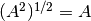does not necessarily hold:

>>> A = matrix([[4,1,4],[7,8,9],[10,2,11]])
>>> sqrtm(A**2)
[ 4.0  1.0   4.0]
[ 7.0  8.0   9.0]
[10.0  2.0  11.0]
>>> sqrtm(A)**2
[ 4.0  1.0   4.0]
[ 7.0  8.0   9.0]
[10.0  2.0  11.0]
>>> A = matrix([[-4,1,4],[7,-8,9],[10,2,11]])
>>> sqrtm(A**2)
[  7.43715112194995  -0.324127569985474   1.8481718827526]
[-0.251549715716942    9.32699765900402  2.48221180985147]
[  4.11609388833616   0.775751877098258   13.017955697342]
>>> chop(sqrtm(A)**2)
[-4.0   1.0   4.0]
[ 7.0  -8.0   9.0]
[10.0   2.0  11.0]


For some matrices, a square root does not exist:

>>> sqrtm([[0,1], [0,0]])
Traceback (most recent call last):
...
ZeroDivisionError: matrix is numerically singular


Two examples from the documentation for Matlab’s sqrtm:

>>> mp.dps = 15; mp.pretty = True
>>> sqrtm([[7,10],[15,22]])
[1.56669890360128  1.74077655955698]
[2.61116483933547  4.17786374293675]
>>>
>>> X = matrix(\
...   [[5,-4,1,0,0],
...   [-4,6,-4,1,0],
...   [1,-4,6,-4,1],
...   [0,1,-4,6,-4],
...   [0,0,1,-4,5]])
>>> Y = matrix(\
...   [[2,-1,-0,-0,-0],
...   [-1,2,-1,0,-0],
...   [0,-1,2,-1,0],
...   [-0,0,-1,2,-1],
...   [-0,-0,-0,-1,2]])
>>> mnorm(sqrtm(X) - Y)
4.53155328326114e-19

mpmath.logm(ctx, A)

Computes a logarithm of the square matrix, i.e. returns a matrix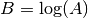such that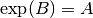. The logarithm of a matrix, if it exists, is not unique.

Examples

Logarithms of some simple matrices:

>>> from mpmath import *
>>> mp.dps = 15; mp.pretty = True
>>> X = eye(3)
>>> logm(X)
[0.0  0.0  0.0]
[0.0  0.0  0.0]
[0.0  0.0  0.0]
>>> logm(2*X)
[0.693147180559945                0.0                0.0]
[              0.0  0.693147180559945                0.0]
[              0.0                0.0  0.693147180559945]
>>> logm(expm(X))
[1.0  0.0  0.0]
[0.0  1.0  0.0]
[0.0  0.0  1.0]


A logarithm of a complex matrix:

>>> X = matrix([[2+j, 1, 3], [1-j, 1-2*j, 1], [-4, -5, j]])
>>> B = logm(X)
>>> nprint(B)
[ (0.808757 + 0.107759j)    (2.20752 + 0.202762j)   (1.07376 - 0.773874j)]
[ (0.905709 - 0.107795j)  (0.0287395 - 0.824993j)  (0.111619 + 0.514272j)]
[(-0.930151 + 0.399512j)   (-2.06266 - 0.674397j)  (0.791552 + 0.519839j)]
>>> chop(expm(B))
[(2.0 + 1.0j)           1.0           3.0]
[(1.0 - 1.0j)  (1.0 - 2.0j)           1.0]
[        -4.0          -5.0  (0.0 + 1.0j)]


A matrix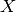close to the identity matrix, for which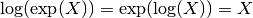holds:

>>> X = eye(3) + hilbert(3)/4
>>> X
[              1.25             0.125  0.0833333333333333]
[             0.125  1.08333333333333              0.0625]
[0.0833333333333333            0.0625                1.05]
>>> logm(expm(X))
[              1.25             0.125  0.0833333333333333]
[             0.125  1.08333333333333              0.0625]
[0.0833333333333333            0.0625                1.05]
>>> expm(logm(X))
[              1.25             0.125  0.0833333333333333]
[             0.125  1.08333333333333              0.0625]
[0.0833333333333333            0.0625                1.05]


A logarithm of a rotation matrix, giving back the angle of the rotation:

>>> t = 3.7
>>> A = matrix([[cos(t),sin(t)],[-sin(t),cos(t)]])
>>> chop(logm(A))
[             0.0  -2.58318530717959]
[2.58318530717959                0.0]
>>> (2*pi-t)
2.58318530717959


For some matrices, a logarithm does not exist:

>>> logm([[1,0], [0,0]])
Traceback (most recent call last):
...
ZeroDivisionError: matrix is numerically singular


Logarithm of a matrix with large entries:

>>> logm(hilbert(3) * 10**20).apply(re)
[ 45.5597513593433  1.27721006042799  0.317662687717978]
[ 1.27721006042799  42.5222778973542   2.24003708791604]
[0.317662687717978  2.24003708791604    42.395212822267]

mpmath.powm(ctx, A, r)

Computes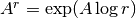for a matrixand complex number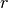.

Examples

Powers and inverse powers of a matrix:

>>> from mpmath import *
>>> mp.dps = 15; mp.pretty = True
>>> A = matrix([[4,1,4],[7,8,9],[10,2,11]])
>>> powm(A, 2)
[ 63.0  20.0   69.0]
[174.0  89.0  199.0]
[164.0  48.0  179.0]
>>> chop(powm(powm(A, 4), 1/4.))
[ 4.0  1.0   4.0]
[ 7.0  8.0   9.0]
[10.0  2.0  11.0]
>>> powm(extraprec(20)(powm)(A, -4), -1/4.)
[ 4.0  1.0   4.0]
[ 7.0  8.0   9.0]
[10.0  2.0  11.0]
>>> chop(powm(powm(A, 1+0.5j), 1/(1+0.5j)))
[ 4.0  1.0   4.0]
[ 7.0  8.0   9.0]
[10.0  2.0  11.0]
>>> powm(extraprec(5)(powm)(A, -1.5), -1/(1.5))
[ 4.0  1.0   4.0]
[ 7.0  8.0   9.0]
[10.0  2.0  11.0]


A Fibonacci-generating matrix:

>>> powm([[1,1],[1,0]], 10)
[89.0  55.0]
[55.0  34.0]
>>> fib(10)
55.0
>>> powm([[1,1],[1,0]], 6.5)
[(16.5166626964253 - 0.0121089837381789j)  (10.2078589271083 + 0.0195927472575932j)]
[(10.2078589271083 + 0.0195927472575932j)  (6.30880376931698 - 0.0317017309957721j)]
>>> (phi**6.5 - (1-phi)**6.5)/sqrt(5)
(10.2078589271083 - 0.0195927472575932j)
>>> powm([[1,1],[1,0]], 6.2)
[ (14.3076953002666 - 0.008222855781077j)  (8.81733464837593 + 0.0133048601383712j)]
[(8.81733464837593 + 0.0133048601383712j)  (5.49036065189071 - 0.0215277159194482j)]
>>> (phi**6.2 - (1-phi)**6.2)/sqrt(5)
(8.81733464837593 - 0.0133048601383712j)